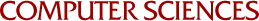Graph Isomorphism for Colored Graphs with Color Multiplicity Bounded by 3

Akash Lal, Dieter van Melkebeek
2005

The colored graph isomorphism problem is a restricted version of the general graph isomorphism (GI)problem that involves deciding the existence of a color preserving isomorphism between a pair of colored graphs. In this report, we study this problem for graphs whose color multiplicity is bounded by 3 (3-GI). We begin by formally defining the colored graph isomorphism problem and setup our notation. Next, we study the general graph isomorphism problem and specialize its results for colored graphs. Then, we use special properties of colored graphs with multiplicity bounded by 3 to prove that 3-GI is in the deterministic-logarithmic-space complexity class L. Finally, we use this result to show that the problem of deciding the existence of a non-trivial color preserving automorphism on a graph with color multiplicity bounded by 3 (3-GA) is in L as well. In previous work , a proof of 3-GI in symmetric logspace SL (which we now know to be the same as L) has been given but the proof is incorrect and section 4 presents a counter example.